CDA数据科学研究院 CDA考试中心 企业服务 关于CDA

#全国校区## python办公自动化｜批量处理文件，一学就会2020-10-15• Python批量读取不同文件夹(⭐⭐⭐)
• Pandas数据处理(⭐⭐)
• Python操作Markdown文件(⭐)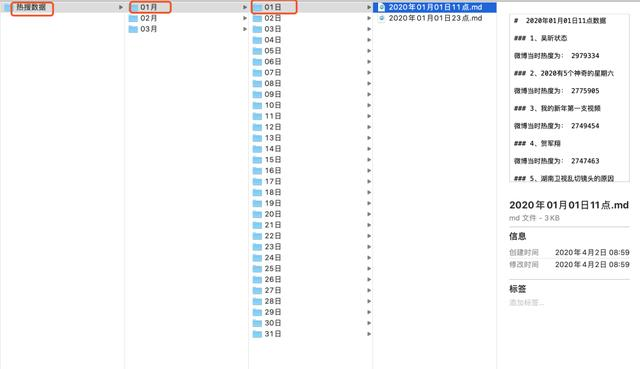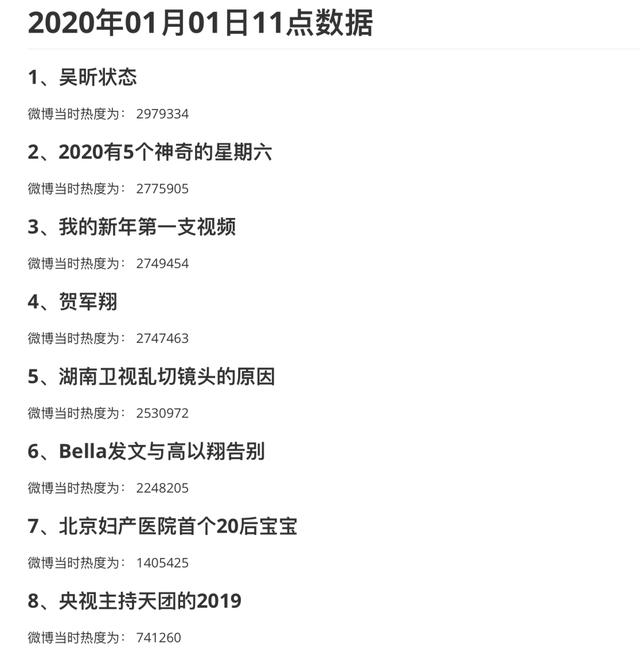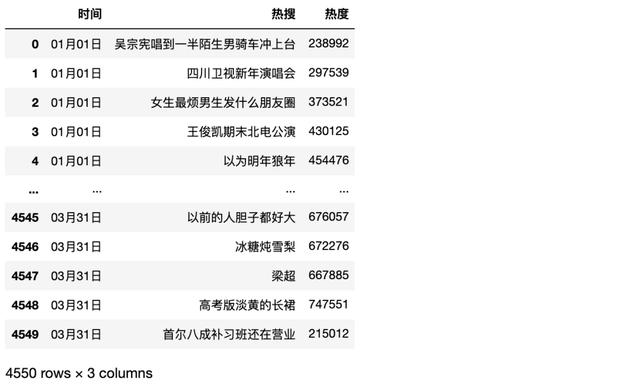Python实现

```import pandas as pd
import os
import glob
from pathlib import Path```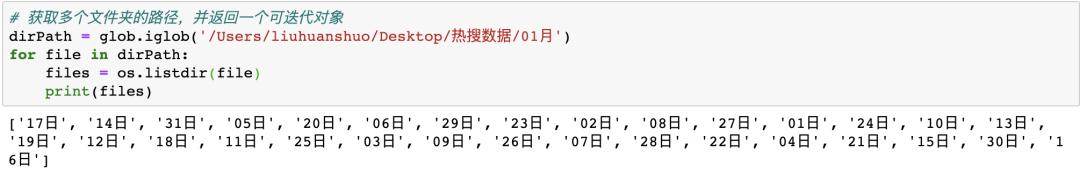```from pathlib import Path

p = Path("/Users/liuhuanshuo/Desktop/热搜数据/") #初始化构造Path对象

FileList=list(p.glob("**/*.md")) #得到所有的markdown文件```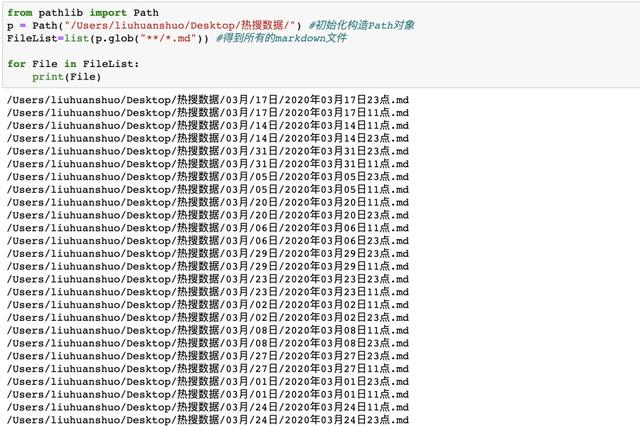`filelist = list(filter(lambda x: str(x).find("23点") >= 0, FileList))`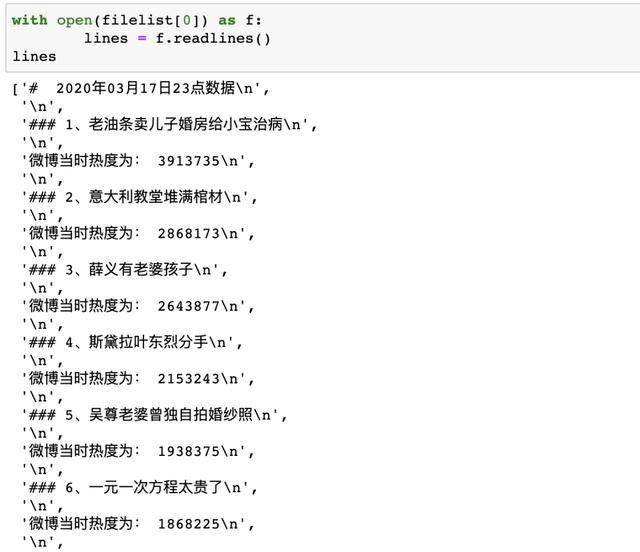```with open(file) as f:
lines = [i.strip() for i in lines] #去除空字符
data = list(filter(None, lines))
del data
data = data[0:100]
date = re.findall('年(.+)2',str(file))
content = data[::2] #奇偶分割
rank = data[1::2]
#提取内容与排名
for i in range(len(content)):
content[i] = re.findall('、(.+)',content[i])
for i in range(len(rank)):
rank[i] = re.findall(' (.+)',rank[i])```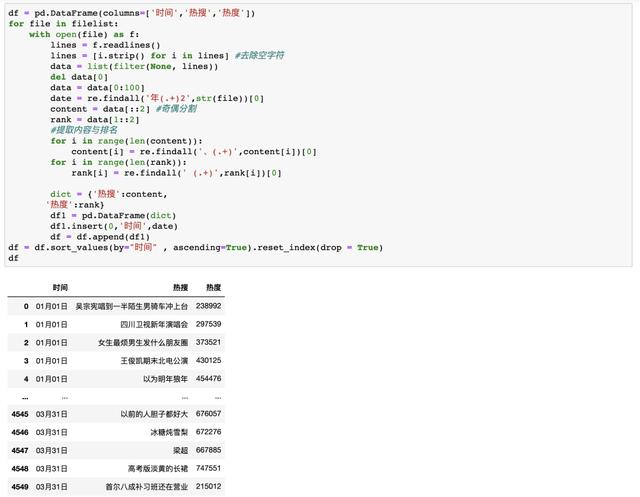OK